Homomorphisms and quotient rings
Isomorphism: Here are the addition and multiplication tables of a ring with two elements, which for now I will call o and i.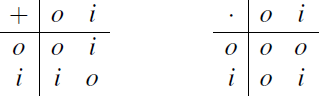You may recognise this ring in various guises: it is the Boolean ringP(X), where X = {x} is a set with just one element x; we have o = /0 and i = {x}. Alternatively
it is the ring of integers mod 2, with o = 2 and i = 2. The fact that these two rings have the same addition and multiplication tables shows that, from an algebraic point of view, we cannot distinguish between them. We formalise this as follows. Let R1 and R2 be rings. Let θ : R1 ! R2 be a function which is one-to-one and onto, that is, a bijection between R1 and R2. Now we denote the result of applying the function q to an element r ∈ R1 by rθ or (r)θ rather than by θ(r); that is, we write the function on the right of its argument. Now we say that θ is an isomorphism from R1 to R2 if it is a bijection which satisfies
(r1 r2)θ = r1θ r2θ, (r1r2)θ = (r1θ)(r2θ). (2.1)
This means that we “match up” elements in R1 with elements in R2 so that addition and multiplication work in the same way in both rings.

Example To return to our earlier example, let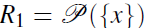and let R2 be the ring of integers mod 2, and define a function θ : R1 -> R2 by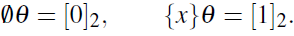Then θ is an isomorphism.

We say that the rings R1 and R2 are “isomorphic” if there is an isomorphism from R1 to R2. The word “isomorphic” means, roughly speaking, “the same shape”: if two rings are isomorphic then they can be regarded as identical from the point of view of Ring Theory, even if their actual elements are quite different (as in our example). We could say that Ring Theory is the study of properties of rings which are the same in isomorphic rings. So, for example, if R1 and R2 are isomorphic then:
• If R1 is commutative, then so is R2, and vice versa; and the same holds for the property of being a ring with identity, a division ring, a Boolean ring, a zero ring, etc.
• However, the property of being a ring of matrices, or a ring of polynomials, etc., are not necessarily shared by isomorphic rings. We use the notation R1 = R2 to mean “R1 is isomorphic to R2”. Remember that isomorphism is a relation between two rings. If you are given two rings R1 and R2 and asked whether they are isomorphic, do not say “R1 is isomorphic but R2 is not”.

Homomorphisms: An isomorphism is a function between rings with two properties: it is a bijection (one-to-one and onto), and it preserves addition and multiplication (as expressed by equation (2.1)). A function which preserves addition and multiplication but is not necessarily a bijection is called a homomorphism. Thus, a homomorphism from R1 to R2 is a function q : R1 -> R2 satisfying
(r1 r2)θ = r1θ r2θ, (r1r2)θ = (r1θ)(r2θ).
You should get used to these two long words, and two others. A function q : R1! R2 is

• a homomorphism if it satisfies (2.1); (homo=similar)
• a monomorphism if it satisfies (2.1) and is one-to-one; (mono=one)
• an epimorphism if it satisfies (2.1) and is onto; (epi=onto)
• an isomorphism if it satisfies (2.1) and is one-to-one and onto (iso=equal)

For example, the function from the ring Z to the ring of integers mod 2, which takes the integer n to its congruence class [n]2 mod 2, is a homomorphism. Basically this says that, if we only care about the parity of an integer, its congruence mod 2, then the addition and multiplication tables are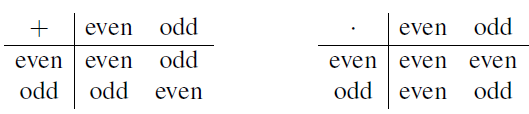and this ring is the same as the one at the start of this section.

Let q : R1 -> R2 be a homomorphism. The image of θ is, as usual, the set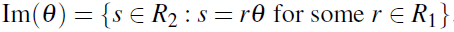We define the kernel of θ to be the set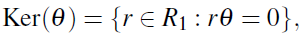the set of elements of R1 which are mapped to the zero element of R2 by q. You will have seen a definition very similar to this in Linear Algebra. The image and kernel of a homomorphism have an extra property. This is not the final version of this theorem: we will strengthen it in two ways in the next two sections. First, a lemma:

Ideals: An ideal in a ring is a special kind of subring.
Let S be a subring of R. We say that S is an ideal if, for any a ∈ S and r ∈ R, we have ar ∈ S and ra ∈ S.
For example, let R = Z and S = nZ for some positive integer n. We know that S is a subring of R. Choose a 2 S, say a = nc for some c ∈ Z. Then ar = ra = n(cr) ∈ S. So S is an ideal. Any ring R has two trivial ideals: the whole ring R is an ideal; and the set {0} consisting only of the zero element is an ideal.